# Texas Go Math Grade 4 Lesson 8.1 Answer Key Multiply by Tens

Refer to our Texas Go Math Grade 4 Answer Key Pdf to score good marks in the exams. Test yourself by practicing the problems from Texas Go Math Grade 4 Lesson 8.1 Answer Key Multiply by Tens.

## Texas Go Math Grade 4 Lesson 8.1 Answer Key Multiply by Tens

Essential Question

What strategies can you use to multiply by tens?
Multiplication is repeated addition. When multiplying whole numbers by 10, simply add a 0 to the end of the number. When multiplying decimals by 10, move the decimal point one space to the right.

Unlock the Problem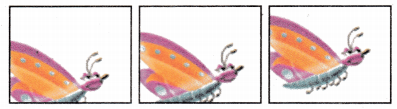Animation for a computer-drawn cartoon requires about 20 frames per second. How many frames would need to he drawn for a 30-second cartoon?
20 x 30 = 600
Explanation:
Multiply by 10 strategy is used.
First multiply the tens place and add zeros to ones place.
So, 20 x 30 = 600
The phrase “20 frames per second’’ means 20 frames are needed for each second of animation. How does this help you know what operation to use?
Multiplication operation is used
20 x 30 = 600
Explanation:
A mathematical operation, symbolized by a × b, a ⋅ b, a ∗ b, or ab, and signifying,
when a and b are positive integers, that a is to be added to itself as many times as there are units in b; the addition of a number to itself as often as is indicated by another number, as in 2×3 or 5×10.

One Way Use place value.

Multiply. 20 × 30
You can think of 30 as 3 tens.
20 × 30 = 20 × __________ tens
= __________ tens
= 600
20 × 30 = 20 ×3 tens
= 60 tens
= 600
Explanation:
Multiplication is repeated addition. When multiplying whole numbers by 10, simply add a 0 to the end of the number. When multiplying decimals by 10, move the decimal point one space to the right.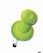Remember
The Associative Property states that you can group factors in different ways and get the same product. Use parentheses to group the factors you multiply first.

Another Way Use the Associative Property.

You can think of 30 as 3 × 10.
20 × 30 = 20 × (3 × 10)
= (20 × 3) × 10
= __________ × __________
= __________
600 frames would need to be drawn.
= (20 × 3) × 10
= 60 × 10
= 600
Explanation:
Associative law, in mathematics, either of two laws relating to number operations of addition and multiplication, stated symbolically: a + (b + c) = (a + b) + c, and a(bc) = (ab)c; that is, the terms or factors may be associated in any way desired.

Math Talk

Mathematical Processes
How can you use place value to tell why 60 × 10 = 600? Explain.
6 x 10 x 10
= 6 x tens x 10
= 600
Explanation:
The order of place value of digits in a number from right to left is ones or units, tens, hundreds, thousands, ten thousand, a hundred thousand, and so on.
6 x 10, in the 6 is in tens place,
So, 10 x 10 x 6 = 600

• Compare the number of zeros in each factor to the number of zeros in the product. What do you notice?
Same number of zeros are noticed.
Explanation:
6 x 10 x 10 there are two zeros
60 x 10 = 600 here also two zeros
So, the number of zeros in each factor to the number of zeros in the product are same.

Another Way

Use mental math to find 14 × 30.

Use the halving-and-doubling strategy.

STEP 1 Find half of 14 to make the problem simpler.

Think: To find half of a number, divide by 2.
14 ÷ 2 = ___________
So, 14 × 30 = 420.
14 ÷ 2 = 7
So, 14 × 30 = 420.
Explanation:
To find half of a number, divide by 2.
14 ÷ 2 = 7
Then multiply with 30
So, 14 × 30 = 420.

STEP Multiply
7 × 30 = ____________
7 × 30 = 210
Explanation:
STEP 3 Double 210.
Multiply 7 with 3 and add 0 in ones pace.

Think: To double a number, multiply by 2.
2 × 210 = ____________
2 x 210 = 420
Explanation:
To double a number, multiply by 2.
2 x 210 = 420

Try This! Multiply.

Use mental math to find 12 × 40.
Explanation:
Mental Math Method
STEP 1 Find half of 12 to make the problem simpler.
12 ÷ 2 = 6
STEP 2 Multiply
6 x 40 = 240
STEP 3 Double 240.
2 x 240 = 480.

Use place value to find 12 × 40.
Place value Method
12 x (4 x 10)
= (12 x 4) x 10
= 48 x 10
= 480
Explanation:
Explanation:
The order of place value of digits in a number from right to left is ones or units, tens, hundreds, thousands, ten thousand, a hundred thousand, and so on.
So, 12 x 40 = 480

Share and Show

Question 1.
Find 20 × 27. Tell which method you chose. Explain what happens in each step. Choose a method. Then find the product.

Mental Math Method
STEP 1 Find half of 20 to make the problem simpler.
20 ÷ 2 = 10
STEP 2 Multiply
10 x 27 = 270
STEP 3 Double 270.
2 x 270 = 540.

Use place value to find 20 × 27.
Place value Method
27 x (2 x 10)
= (27 x 2) x 10
= 54 x 10
= 540
Explanation:
When multiplying whole numbers by 10, simply
add a 0 to the end of the number.
The order of place value of digits in a number from right to left is ones or units, tens, hundreds, thousands, ten thousand, a hundred thousand, and so on.

Choose a method. Then find the product.

Question 2.
10 × 12
Explanation:
Mental Math Method
STEP 1 Find half of 12 to make the problem simpler.
12 ÷ 2 = 6
STEP 2 Multiply
6 x 10 = 60
STEP 3 Double 60.
2 x 60 = 120.

Question 3.
20 × 20
Explanation:
Mental Math Method
STEP 1 Find half of 20 to make the problem simpler.
20 ÷ 2 = 10
STEP 2 Multiply
10 x 10 = 100
STEP 3 Double 200
2 x 200 = 400.

Question 4.
40 × 24
Explanation:
Mental Math Method
STEP 1 Find half of 24 to make the problem simpler.
24 ÷ 2 = 12
STEP 2 Multiply
12 x 10 = 120
STEP 3 Double 120.
2 x 120 = 240
STEP 4 Double 240.
240 x 2 = 480

Question 5.
11 × 60
Explanation:
Mental Math Method
STEP 1 Find half of 60 to make the problem simpler.
60 ÷ 2 = 30
STEP 2 Multiply
30 x 10 = 300
STEP 3 Double 300.
2 x 300 = 600.
600 + 60 = 660

Problem Solving

H.O.T Algebra Find the unknown digit in the number.

Question 6.
64 × 40 = 2,56= __________
64 x 4 x 10
256 x 10
2560= 0
Explanation:
To find unknown multiply terms, multiply the coefficients and add the exponents on each variable.
The number of terms in the product will be equal to the product of the number of terms.

Question 7.
29 × 50 = 1,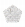50= ____________
29 x 50
=29 x 5 x 10
=145 x 10
=1450= 4
Explanation:
To find unknown multiply terms, multiply the coefficients and add the exponents on each variable.
The number of terms in the product will be equal to the product of the number of terms.

Question 8.
3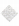× 47 = 1,410= _____________
47 x 3
141
141_  = 1410= 0
Explanation:
To find unknown multiply terms, multiply the coefficients and add the exponents on each variable.
The number of terms in the product will be equal to the product of the number of terms.

Problem solving

Use the table for 9-10.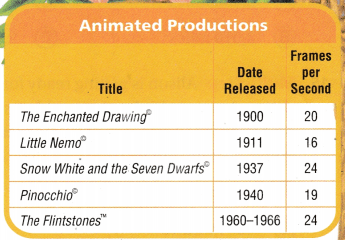Question 9.
How many frames did it take to produce 50 seconds of Pinocchio?
950 frames for 50 seconds.
Explanation:
Pinocchio 19 seconds to produce 1 frame.
So, to produce 50 seconds
50 x 19
= 19 x 5 x 10
= 95 x 10
= 950 frames for 50 seconds

Question 10.
Are there fewer frames in 10 seconds of The Flintstones or in 14 seconds of The Enchanted Drawing? What is the difference in the number of frames?
The Flintstones 40 frames less then The Enhanced Drawing.
Explanation:
The Flintstones         The Enhanced Drawing
10 x 24 =  240                       14 x 20 = 280
24 tens                                   28 tens
The Flintstones 40 frames less then The Enhanced Drawing

Question 11.H.O.T. The product of my number and twice my number is 128. What is half my number? Explain how you solved the problem.
Half of my number is 64
Explanation:
let my number is X
twice my number is  2X
X
x 2(X) = 128
X x (X) = 128/2
X x (X) = 64
X = 8           8 x 8 = 68
my number is 8
X x 2(X) = 128
8 x 2 x (8) = 8 x 16 = 128

Question 12.
H.O.T. Multi-Step What’s the Error? Tanya says that the product of a multiple often and a multiple of ten will always have only one zero. Is she correct? Explain.
Explanation:
only one zero is not correct
Examples,
10 x 10 = 100
10 x 2 = 20
3 x 10 = 30

Question 13.
A giant kelp plant can grow 60 centimetres per day. About how much can it grow in 30 days?
(A) 180 centimetres
(B) 18,000 centimetres
(C) 1,800 centimetres
(D) 1,200 centimetres
Option (C)
Explanation:
A giant kelp plant can grow 60 centimetres per day.
About how much can it grow in 30 days
60 x 30
(6 x 3) (10 x 10)
18 x 100
1800 centimetres

Question 14.
Mrs. Sanchez drives a total of 50 miles for work each day.
How many miles does she drive in 42 days?
(A) 2,000 miles
(B) 2,010 miles
(C) 210 miles
(D) 2,100 miles
Option(D)
Explanation:
Mrs. Sanchez drives a total of 50 miles for work each day.
Number of miles she drive in 42 days
50 x 42 =2,100 miles

Question 15.
Multi-Step Alison is getting ready for a math contest. Each day, she works on multiplication problems for 20 minutes and division problems for 10 minutes. How many minutes does Alison practice multiplication and division problems in 15 days?
(A) 300 minutes
(B) 150 minutes
(C) 350 minutes
(D) 450 minutes
Option(D)
Explanation:
Each day, Alison works on multiplication problems for 20 minutes and
works on division problems for 10 minutes.
Each day she works for 10 + 20 = 30
Number of minutes Alison practice multiplication and division problems in 15 days
30 x 15 = 450 minutes

TEXAS Test Prep

Question 16.
Luis jogs 10 miles a week. He bikes 20 miles a week. How far will he have jogged in 26 weeks?
(A) 30 miles
(B) 200 miles
(C) 260 miles
(D) 520 miles
Option(C)
Explanation:
Luis jogs 10 miles a week.
He bikes 20 miles a week.
Number of mile he jogged in 26 weeks
26 x 10 = 260 miles.

### Texas Go Math Grade 4 Lesson 8.1 Homework and Practice Answer Key

Choose a method. Then find the product.

Question 1.
30 × 40 = __________
Explanation:
Mental Math Method
STEP 1 Find half of 30 to make the problem simpler.
30 ÷ 2 = 15
STEP 2 Multiply
15 x 40 = 600
STEP 3 Double 600.
2 x 600 = 1200.

Use place value to find 30 × 40.
Place value Method
30 x (4 x 10)
= (30 x 4) x 10
= 120 x 10
= 1200
Explanation:
The order of place value of digits in a number from right to left is ones or units, tens, hundreds, thousands, ten thousand, a hundred thousand, and so on.
So, 30 x 40 = 1200

Question 2.
16 × 20 = __________
Explanation:
Mental Math Method
STEP 1 Find half of 16 to make the problem simpler.
16 ÷ 2 = 8
STEP 2 Multiply
8 x 2 = 16
STEP 3 Double 16.
2 x 16 = 32.
Multiply with 10
32 x 10 = 320

Question 3.
50 × 11 = __________
Explanation:
Place value Method
11 x (5 x 10)
= (11 x 5) x 10
= 55 x 10
= 550
Explanation:
The order of place value of digits in a number from right to left is ones or units, tens, hundreds, thousands, ten thousand, a hundred thousand, and so on.
So, 50 x 11= 550

Find the unknown digit in the number.

Question 4.
54 × 30 = 162= __________
Explanation:
54 x 30
54 x 3 x 10
162 x 10
1620
1620 = 162= 0

Question 5.
36 × 40 = 140= ____________
Explanation:
36 x 40
36 x 4 x 10
144 x 10 = 1440= 4

Question 6.
2× 62 = 1,240=_____
62 x 2
124
124_ = 1240= 0

Problem Solving

Use the table for 7-8.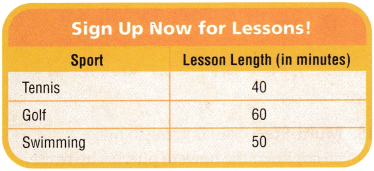Question 7.
The tennis instructor teaches 20 lessons each week. How many minutes does she teach?
800 minutes
Explanation:
The tennis instructor teaches 20 lessons each week.
Total minutes she teach
20 x 40
20 x 4 x 10
80 x 10 =800 minutes

Question 8.
Last month, the golf teacher taught 32 lessons. The swimming teacher taught 40 lessons. Which teacher taught for the most minutes? What is the difference in the number of minutes?
Swimming teacher taught more minutes, the difference is 80 minutes
Explanation:
i) golf teacher taught 32 lessons
32 x 60
32 x 6 x 10
192 x 10 = 1920
ii) swimming teacher taught 40 lessons
40 x 50
40 x 5 x 10
200 x 10 = 2000
the difference in the number of minutes is 2000 – 1920 = 80 minutes
Swimming teacher taught more minutes

Question 9.
The product of half my number times my number is 162. What is twice my number? Explain how you solved the problem.
Explanation:
let my number is X
half my number is  X/2
X
x (X/2) = 162
X x (X) = 162 x 2
X x (X) = 324                 (18 x 18 = 324)
number is X = 18
twice my number 2 X = 2 x 18 = 36

Lesson Check

Question 10.
Julian practices his trombone 30 minutes each day. How many minutes does he practice in 31 days?
(A) 1,200 minutes
(B) 610 minutes
(C) 930 minutes
(D) 630 minutes
Explanation:
Julian practices his trombone 30 minutes each day.
Number of minutes he practice in 31 days
30 x 31
31 x 3 x 10
93 x 10 = 930

Question 11.
There are 50 lollipops in a bag. How many lollipops are there in 12 bags?
(A) 600
(B) 512
(C) 1,100
(D) 520
Option(A)
Explanation:
There are 50 lollipops in a bag.
Number of lollipops are there in 12 bags
50 x 12 = 600

Question 12.
A factory makes 80 bicycles a day. How many bicycles do they make in 22 days?
(A) 320
(B) 1,760
(C) 1,660
(D) 844
Option(B)
Explanation:
A factory makes 80 bicycles a day.
Number of bicycles they make in 22 days
80 x 22 = 1,760

Question 13.
Mala has 20 vases. She puts 12 flowers in each vase. How many flowers does she use?
(A) 24
(B) 144
(C) 440
(D) 240
Option(D)
Mala has 20 vases
She puts 12 flowers in each vase.
Number of flowers she use
20 x 12 = 240

Question 14.
Multi-Step Caroline packs 12 jars of jam in a box. She has 40 boxes. She has 542 jars of jam. How many jars of jam will she have left when all the boxes are full?
(A) 62
(B) 42
(C) 52
(D) 72
Option(A)
Explanation:
Caroline packs 12 jars of jam in a box.
She has 40 boxes.
So, 40 x 12 = 480
She packs 480 jar jams in 40 boxes.
She has 542 jars of jam.
Total jars of jam left with her when all the boxes are full.
542 – 480 = 62

Question 15.
Multi-Step Sam is going to make 30 copies of the book of puzzles he wrote. The book has 18 pages. He has 500 sheets of paper. How many more sheets of paper does he need?
(A) 30
(B) 140
(C) 40
(D) 130